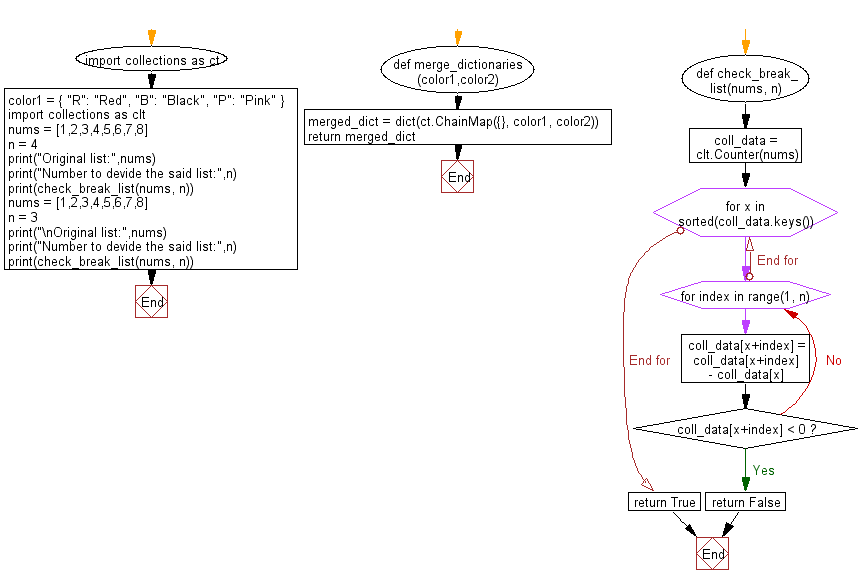﻿ Python: Break a list of integers into sets of a given positive number - w3resource# Python: Break a list of integers into sets of a given positive number

## Python Collections: Exercise-19 with Solution

Write a Python program to break a given list of integers into sets of a given positive number. Return true or false.

Sample Solution:

Python Code:

``````import collections as clt
def check_break_list(nums, n):
coll_data = clt.Counter(nums)
for x in sorted(coll_data.keys()):
for index in range(1, n):
coll_data[x+index] = coll_data[x+index]  - coll_data[x]
if coll_data[x+index] < 0:
return False
return True

nums = [1,2,3,4,5,6,7,8]
n = 4
print("Original list:",nums)
print("Number to devide the said list:",n)
print(check_break_list(nums, n))
nums = [1,2,3,4,5,6,7,8]
n = 3
print("\nOriginal list:",nums)
print("Number to devide the said list:",n)
print(check_break_list(nums, n))
```
```

Sample Output:

```Original list: [1, 2, 3, 4, 5, 6, 7, 8]
Number to devide the said list: 4
True

Original list: [1, 2, 3, 4, 5, 6, 7, 8]
Number to devide the said list: 3
False
```

Flowchart:## Visualize Python code execution:

The following tool visualize what the computer is doing step-by-step as it executes the said program:

Python Code Editor:

Have another way to solve this solution? Contribute your code (and comments) through Disqus.

What is the difficulty level of this exercise?

Test your Programming skills with w3resource's quiz.

﻿

## Python: Tips of the Day

Use Reversed() In for Loops:

```>>> tasks = ['laundry', 'picking up kids', 'gardening', 'cooking']